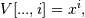# numpy.polynomial.polynomial.polyvander¶

numpy.polynomial.polynomial.polyvander(x, deg)[source]

Vandermonde matrix of given degree.

Returns the Vandermonde matrix of degree deg and sample points x. The Vandermonde matrix is defined bywhere 0 <= i <= deg. The leading indices of V index the elements of x and the last index is the power of x.

If c is a 1-D array of coefficients of length n + 1 and V is the matrix V = polyvander(x, n), then np.dot(V, c) and polyval(x, c) are the same up to roundoff. This equivalence is useful both for least squares fitting and for the evaluation of a large number of polynomials of the same degree and sample points.

Parameters: x : array_like Array of points. The dtype is converted to float64 or complex128 depending on whether any of the elements are complex. If x is scalar it is converted to a 1-D array. deg : int Degree of the resulting matrix. vander : ndarray. The Vandermonde matrix. The shape of the returned matrix is x.shape + (deg + 1,), where the last index is the power of x. The dtype will be the same as the converted x.